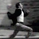# Vertical Horizontal Filter VHF by KIVANÇ fr3762

3852 görüntülenme
Vertical Horizontal Filter
Vertical Horizontal Filter (VHF) was created by Adam White to identify trending and ranging markets. VHF measures the level of trend activity, similar to ADX in the Directional Movement System. Trend indicators can then be employed in trending markets and momentum indicators in ranging markets.

Vary the number of periods in the Vertical Horizontal Filter to suit different time frames. White originally recommended 28 days but now prefers an 18-day window smoothed with a 6-day moving average.

Vertical Horizontal Filter does not, itself, generate trading signals, but determines whether signals are taken from trend or momentum indicators.

Rising values indicate a trend.
Falling values indicate a ranging market.
High values precede the end of a trend.
Low values precede a trend start.

I have added an option to plot a deafult value of 14 bar EMA too, to clarify the signals.

Formula
To calculate the Vertical Horizontal Filter:

Select the number of periods (n) to include in the indicator. This should be based on the length of the cycle that you are analyzing. The most popular is 28 days (for intermediate cycles).
Determine the highest closing price ( HCP ) in n periods.
Determine the lowest closing price (LCP) in n periods.
Calculate the range of closing prices in n periods:
HCP - LCP
Next, calculate the movement in closing price for each period:
Closing price - Closing price
Add up all price movements for n periods, disregarding whether they are up or down:
Sum of absolute values of ( Close - Close ) for n periods
Divide Step 4 by Step 6:
VHF = ( HCP - LCP) / (Sum of absolute values for n periods)